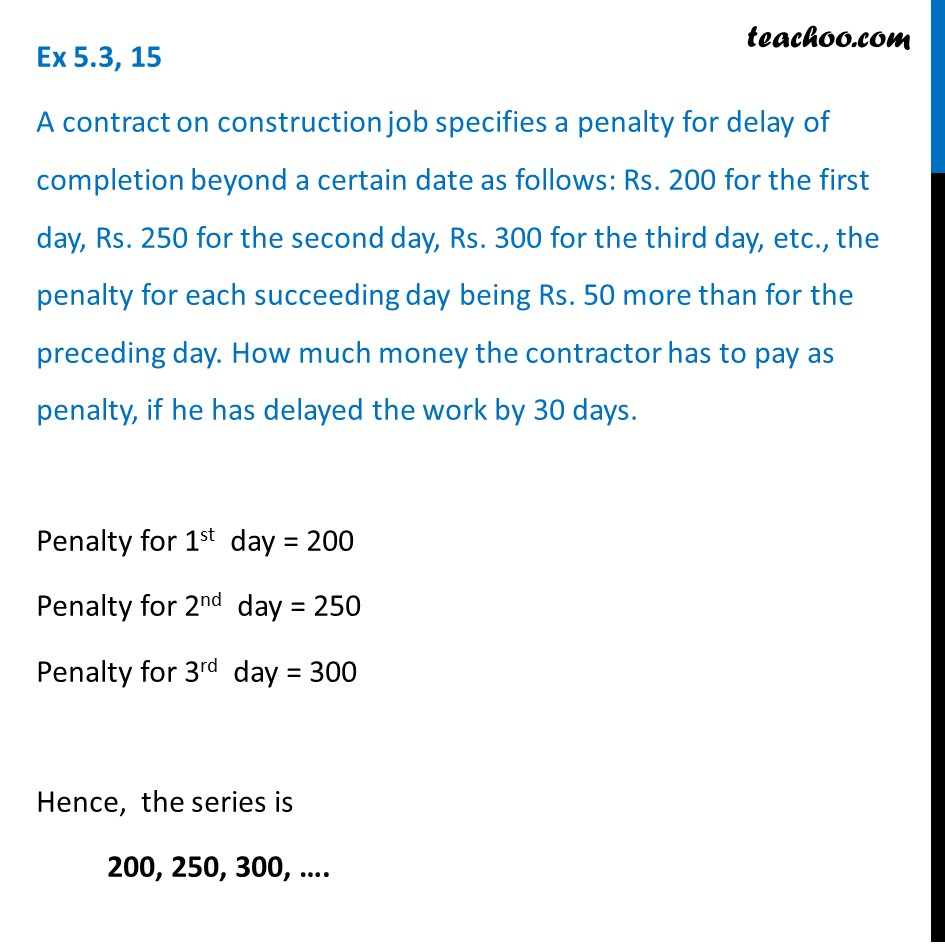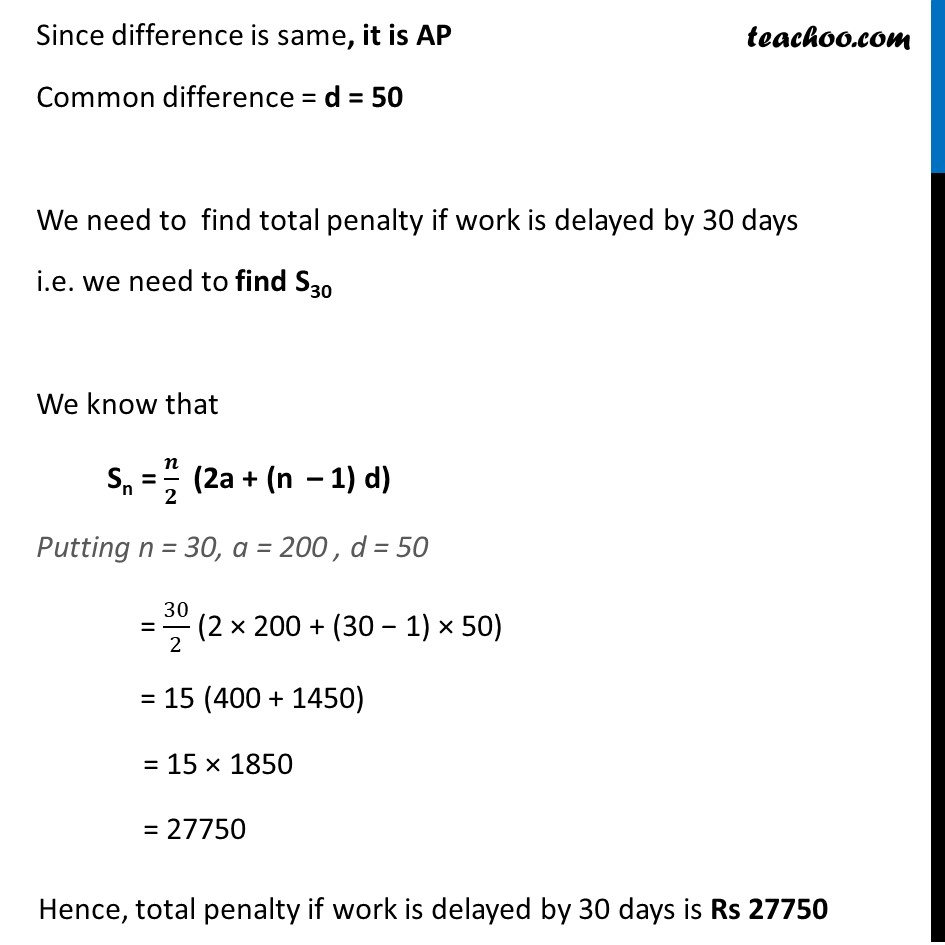Ex 5.3

Chapter 5 Class 10 Arithmetic Progressions
Serial order wiseLearn in your speed, with individual attention - Teachoo Maths 1-on-1 Class

### Transcript

Ex 5.3, 15 A contract on construction job specifies a penalty for delay of completion beyond a certain date as follows: Rs. 200 for the first day, Rs. 250 for the second day, Rs. 300 for the third day, etc., the penalty for each succeeding day being Rs. 50 more than for the preceding day. How much money the contractor has to pay as penalty, if he has delayed the work by 30 days. Penalty for 1st day = 200 Penalty for 2nd day = 250 Penalty for 3rd day = 300 Hence, the series is 200, 250, 300, …. Since difference is same, it is AP Common difference = d = 50 We need to find total penalty if work is delayed by 30 days i.e. we need to find S30 We know that Sn = 𝒏/𝟐 (2a + (n – 1) d) Putting n = 30, a = 200 , d = 50 = 30/2 (2 × 200 + (30 − 1) × 50) = 15 (400 + 1450) = 15 × 1850 = 27750 Hence, total penalty if work is delayed by 30 days is Rs 27750